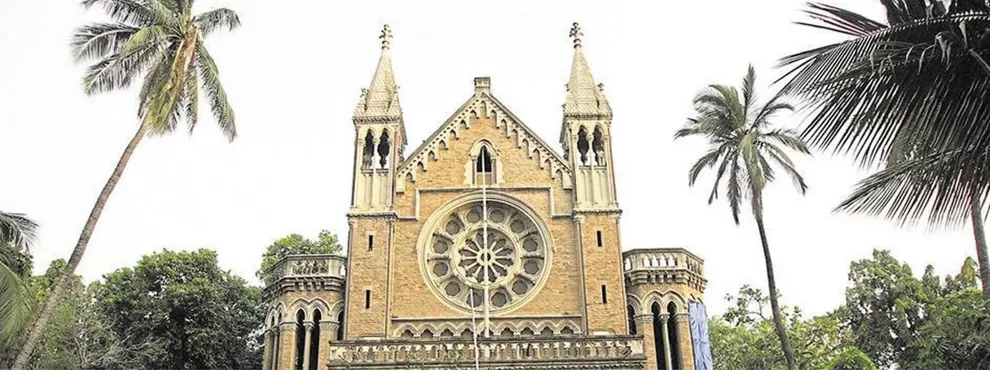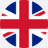# Mumbai University CGPA to Percentage ConverterEdvoy
Last updated: Mar 10, 20238 min readGot a 6 CGPA and higher and planning to study abroad? Try our course finder.

Mumbai University CGPA to Percentage Converter has made it pretty simple and fast to convert Mumbai University CGPA to percentage. No mathematical calculations are needed; enter your CGPA and get the result instantly.

In this guide, we will discuss what CGPA is, how to calculate it, and how you can convert Mumbai University CGPA to percentage using the formula and the converter.

The University of Mumbai is one of the oldest and premier universities in India, having 56 Departments, 12 specialised Centers, 781 Affiliated Colleges, 2 main Campuses, 2 sub Campuses, and 2 Model Colleges.

The university also has its own Engineering College, the School of Engineering and Applied Sciences, at Kalyan. It is spread over an area of 600 km, stretching from Thane district to Sindhudurg district.

In 2001, the University of Mumbai got a 5-star status, and in April 2012 got an ‘A’ grade status from the National Assessment and Accreditation Council (NAAC). It has been ranked at 71st position by the National Institutional Ranking Framework (NIRF).

## What is CGPA?

The Cumulative Grade Point Average or CGPA is a student's overall academic performance. It is the weighted average of the grade points obtained by the student during the whole course since the first semester. Although evaluation criteria differ from country to country, the CGPA system is one of the most widely used evaluation methods.

## How to calculate CGPA in Mumbai University

The CGPA in Mumbai University is calculated as,

CGPA = ∑ (𝐶𝑖 ∗ 𝐺𝑖) / ∑ 𝐶𝑖

where, 𝐶𝑖 are the credits earned in each course, and 𝐺𝑖 are grade points in these courses.

## Grading system of Mumbai University

From the academic year 2016-2017, the Choice Based Credit and Grading System were effective for the Faculty of Technology of the University of Mumbai. The students are awarded a letter grade in every course, which indicates a qualitative assessment of the student’s performance and also carries a quantitative equivalent called the Grade Point.

The letter grade AB will be awarded to the students who are absent in the examination, and the corresponding grade point assigned will be zero.

Related article: How to calculate percentage marks?

## How to convert CGPA to percentage Mumbai University

The university constituted a committee to prepare a formula to convert CGPA to a percentage. On 27th May 2017, the committee passed the following resolutions:

For the 7 Point Grading system (CBGS), the percentage will be calculated on actual marks obtained by the students.

For the 10 Point Grading system (CBCS), the percentage will be calculated according to the following formula:

Percentage = 7.1*CGPA + 11

## CGPA to Percentage Calculator

Got a 6 CGPA and higher and planning to study abroad? Try our course finder.

## Process to calculate CGPA

It is a pretty simple process to calculate the CGPA. Just follow the below 2 steps.

First, calculate the Semester Grade Performance Index (SGPI) for each semester. SGPI is a candidate's performance in a semester. It is the average of grade points obtained in all the courses by the student during the semester. For example, if a student passes five courses in a semester with credits C1, C2, C3, C4, and C5 and obtains G1, G2 , G3, G4 , and G5 grade points respectively, then

SGPI = C1G1 + C2G2 + C3G3 + C4G4 + C5G5 / C1 + C2 + C3 + C4 + C5

Second, add the SGPI of all semesters and divide by the total number of semesters to get the CGPA, so

CGPA = ∑ (SGPI) / Number of semesters

For example, there are 8 semesters in an Engineering course, so

CGPA = ∑ (SGPI) / 8

CGPI to Percentage Conversion Formula for Ten Point scale in facility of Technology of University of Mumbai

Percentage = 7.1*CGPI+12; If CGPI is less than 7
Percentage = 7.4*CGPI+12; If CGPI is greater then and equal to

The percentage can be calculated using above formulae and rounded to Next full integer.

Related Calculators

## Mumbai University CGPA to percentage conversion table

In Mumbai University, CGPA is converted into percentage using the Mumbai University CGPA to percentage conversion formula, Percentage = 7.1 * CGPA + 11. Below is the Mumbai University CGPA to percentage conversion table.

Related pages,

## FAQs

1. What percentage is 10 CGPA in Mumbai University?

To convert CGPA to percentage in Mumbai University, use the Mumbai University CGPA to percentage conversion formula, Percentage = 7.1*CGPA + 11. So, in this case, Percentage = 7.1*10 + 11 = 82

2. What percentage is 7.6 CGPA?

To convert CGPA to percentage in Mumbai University, use the Mumbai University CGPA to percentage conversion formula, Percentage = 7.1*CGPA + 11. So, in this case, Percentage = 7.1*7.6 + 11 = 65

3. How much is 8.2 CGPA in percentage?

To convert CGPA to percentage in Mumbai University, use the Mumbai University CGPA to percentage conversion formula, Percentage = 7.1*CGPA + 11. So, in this case, Percentage = 7.1*8.2 + 11 = 69.2

4. What percentage is 9.25 CGPA?

To convert CGPA to percentage in Mumbai University, use the Mumbai University CGPA to percentage conversion formula, Percentage = 7.1*CGPA + 11. So, in this case, Percentage = 7.1*9.25 + 11 = 76.8

5. What percentage is 7.75 CGPA?

To convert CGPA to percentage in Mumbai University, use the Mumbai University CGPA to percentage conversion formula, Percentage = 7.1*CGPA + 11. So, in this case, Percentage = 7.1*7.75 + 11 = 66

Book for a Remote Counselling to get started!

0 LikesWritten By
Edvoy